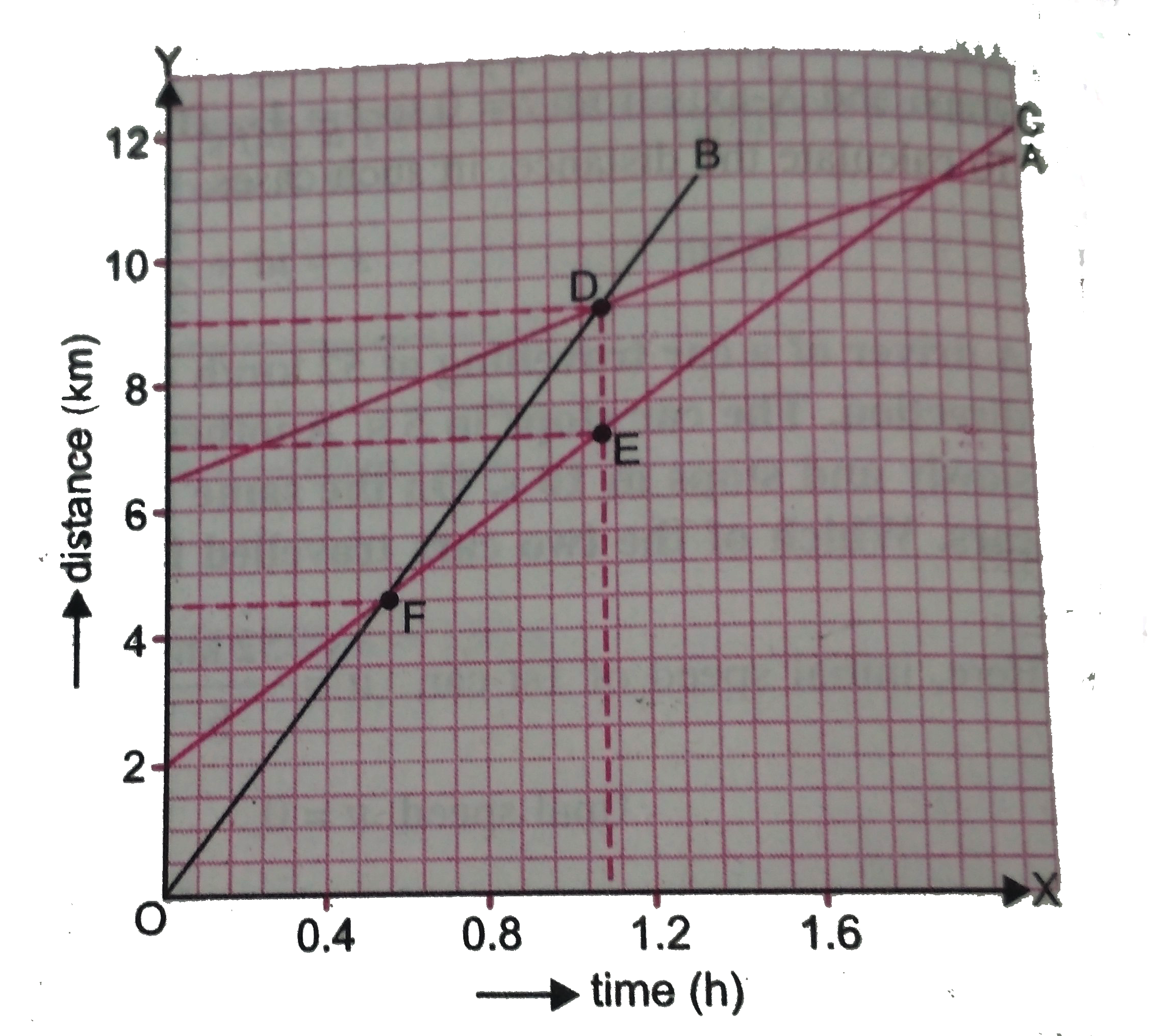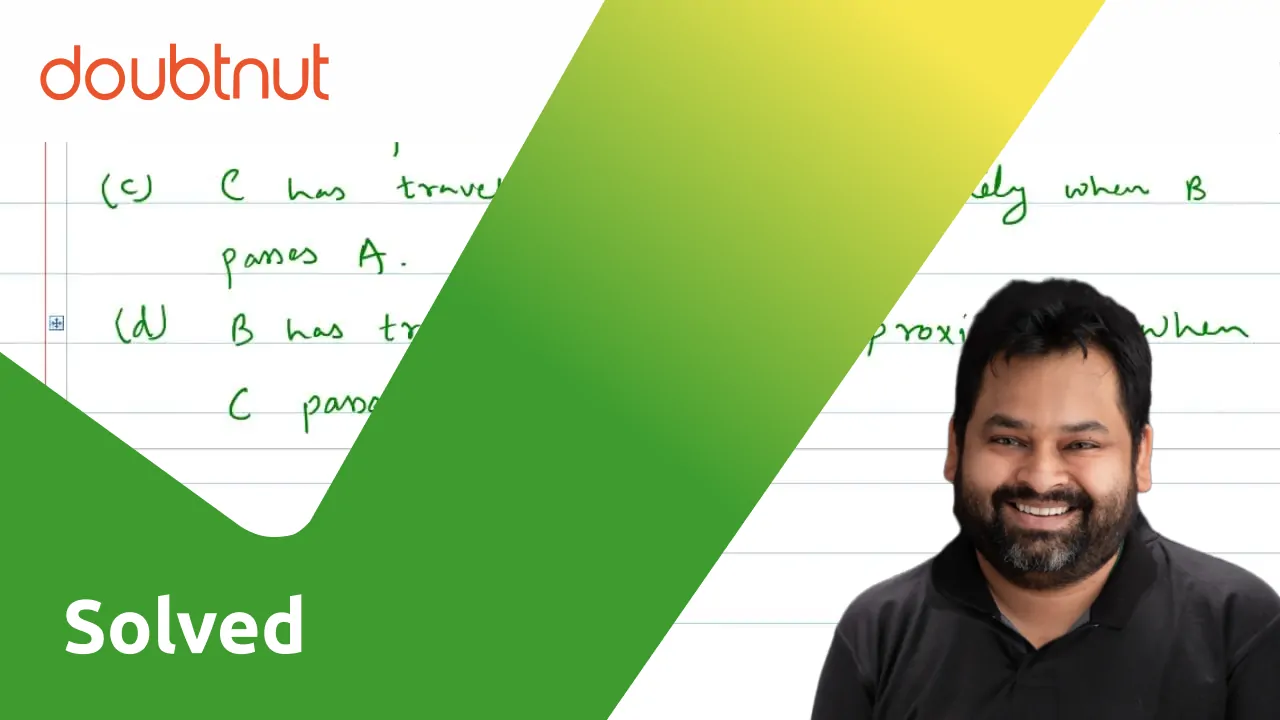Doubtnut App - Get 50 Lakh+ video solutions better than GoogleInstall Now

# Figure shows the distance-time graph of three object A,B and C. Study the graph and answer the following questions : (a) Which of the three is travelling the fastest ? (b) Are all three ever at the same point on the road ? ( c) How far has C travelld by when B passes A ? (d) How far has B travelled by the time it passes C ?

Text Solution
Verified by Experts

## (a) As slope of distance-time graph represents speed of an object, therefore, as is clear from Figure, B has maximum speed, i.e., the object B moves the fastest. (b) For meeting at any point on the road, the respective distance and time values must be same for all the three objects at that point. As th three lines do not meet at a single point, the three objects never meet on the raod. ( c) As is clear from Figure, B passes A at D.At this time, C is at E, which corresponds to 7km. hence when B crosses A, then C is at 7km from the origin. (d) B crossed C at F at a distance of 4.5km from the origin.